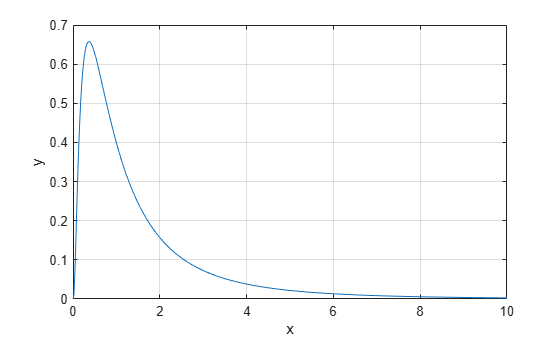lognpdf

Lognormal probability density function

Description

y = lognpdf(x) returns the probability density function (pdf) of the standard lognormal distribution, evaluated at the values in x. In the standard lognormal distribution, the mean and standard deviation of logarithmic values are 0 and 1, respectively.

y = lognpdf(x,mu) returns the pdf of the lognormal distribution with the distribution parameters mu (mean of logarithmic values) and 1 (standard deviation of logarithmic values), evaluated at the values in x.

example

y = lognpdf(x,mu,sigma) returns the pdf of the lognormal distribution with the distribution parameters mu (mean of logarithmic values) and sigma (standard deviation of logarithmic values), evaluated at the values in x.

Examples

collapse all

Compute the pdf values evaluated at the values in x for the lognormal distribution with mean mu and standard deviation sigma.

x = 0:0.02:10;
mu = 0;
sigma = 1;
y = lognpdf(x,mu,sigma);

Plot the pdf.

plot(x,y)
grid on
xlabel('x')
ylabel('y')Input Arguments

collapse all

Values at which to evaluate the pdf, specified as a positive scalar value or an array of positive scalar values.

To evaluate the pdf at multiple values, specify x using an array. To evaluate the pdfs of multiple distributions, specify mu and sigma using arrays. If one or more of the input arguments x, mu, and sigma are arrays, then the array sizes must be the same. In this case, lognpdf expands each scalar input into a constant array of the same size as the array inputs. Each element in y is the pdf value of the distribution specified by the corresponding elements in mu and sigma, evaluated at the corresponding element in x.

Example: [-1,0,3,4]

Data Types: single | double

Mean of logarithmic values for the lognormal distribution, specified as a scalar value or an array of scalar values.

To evaluate the pdf at multiple values, specify x using an array. To evaluate the pdfs of multiple distributions, specify mu and sigma using arrays. If one or more of the input arguments x, mu, and sigma are arrays, then the array sizes must be the same. In this case, lognpdf expands each scalar input into a constant array of the same size as the array inputs. Each element in y is the pdf value of the distribution specified by the corresponding elements in mu and sigma, evaluated at the corresponding element in x.

Example: [0 1 2; 0 1 2]

Data Types: single | double

Standard deviation of logarithmic values for the lognormal distribution, specified as a positive scalar value or an array of positive scalar values.

To evaluate the pdf at multiple values, specify x using an array. To evaluate the pdfs of multiple distributions, specify mu and sigma using arrays. If one or more of the input arguments x, mu, and sigma are arrays, then the array sizes must be the same. In this case, lognpdf expands each scalar input into a constant array of the same size as the array inputs. Each element in y is the pdf value of the distribution specified by the corresponding elements in mu and sigma, evaluated at the corresponding element in x.

Example: [1 1 1; 2 2 2]

Data Types: single | double

Output Arguments

collapse all

pdf values, evaluated at the values in x, returned as a scalar value or an array of scalar values. y is the same size as x, mu, and sigma after any necessary scalar expansion. Each element in y is the pdf value of the distribution specified by the corresponding elements in mu and sigma, evaluated at the corresponding element in x.

collapse all

Lognormal Distribution

The lognormal distribution is a probability distribution whose logarithm has a normal distribution.

The probability density function (pdf) of the lognormal distribution is

$y=f\left(x|\mu ,\sigma \right)=\frac{1}{x\sigma \sqrt{2\pi }}\mathrm{exp}\left\{\frac{-{\left(\mathrm{log}x-\mu \right)}^{2}}{2{\sigma }^{2}}\right\},\text{ }\text{for}\text{\hspace{0.17em}}x>0.$

Alternative Functionality

• lognpdf is a function specific to lognormal distribution. Statistics and Machine Learning Toolbox™ also offers the generic function pdf, which supports various probability distributions. To use pdf, create a LognormalDistribution probability distribution object and pass the object as an input argument or specify the probability distribution name and its parameters. Note that the distribution-specific function lognpdf is faster than the generic function pdf.

• Use the Probability Distribution Function app to create an interactive plot of the cumulative distribution function (cdf) or probability density function (pdf) for a probability distribution.

 Mood, A. M., F. A. Graybill, and D. C. Boes. Introduction to the Theory of Statistics. 3rd ed., New York: McGraw-Hill, 1974. pp. 540–541.

 Evans, M., N. Hastings, and B. Peacock. Statistical Distributions. 2nd ed., Hoboken, NJ: John Wiley & Sons, Inc., 1993.